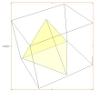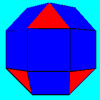#### You may also like### A Mean Tetrahedron

Can you number the vertices, edges and faces of a tetrahedron so that the number on each edge is the mean of the numbers on the adjacent vertices and the mean of the numbers on the adjacent faces?### Rhombicubocts

Each of these solids is made up with 3 squares and a triangle around each vertex. Each has a total of 18 square faces and 8 faces that are equilateral triangles. How many faces, edges and vertices does each solid have?### Icosian Game

This problem is about investigating whether it is possible to start at one vertex of a platonic solid and visit every other vertex once only returning to the vertex you started at.

# Triangles to Tetrahedra

### Why do this problem?

This problem involves using practical equipment to approach a mathematical problem.
It challenges the usual misconception that all tetrahedra are regular.
It needs systematic thinking and visualisation and has some surprises in it - there are a few examples that are quite unexpected.
It is hard to be convinced that you have found all the possibilities and difficult to make the distinction between the two tetrahedra that are mirror images of one another.

### Possible approach

Hand out one of each type of triangle to students working in pairs or small groups. Invite them to make a list of four things that they think are mathematically most important about the triangles - either by considering each triangle individually or when compared to each other.
Share ideas, making sure these points are covered:
• two are isoceles and the other two are equilateral
• one triangle has a right angle
• the triangles have sides of only two possible lengths.
Spend some time comparing the triangles to establish which sides are "short" and which "long".

Hand out further triangles and invite the students to create a tetrahedron with some of the triangles. This task may result in the need to discuss what a tetrahedron is and that a tetrahedron can be made from triangles that are not equilateral. You may wish to have some examples ready to illustrate these points.

Present the problem.

Whilst the class works on the problem it may be useful to stop to discuss progress and approaches that will enable them to convince themselves and each other that they have all the possibilities.

### Key questions

If you take one of each of the triangles is it possible to make a tetrahedron and how do you know?
Have you got a systematic approach for finding all the different tetrahedra?
How are you recording your findings?
How do you know that you have tried all possible ways of putting the same four triangles together?

### Possible support

Work with two and then three different types of triangle to establish a systematic approach.

### Possible extension

Students who have met Pythagoras' Theorem may like to quantify the relationship between long and short sides.
You might encourage students to try the problem Tetrahedra tester